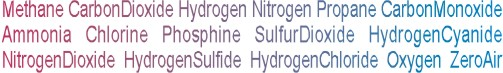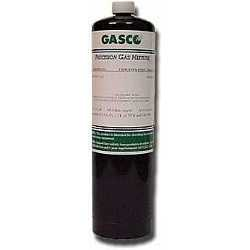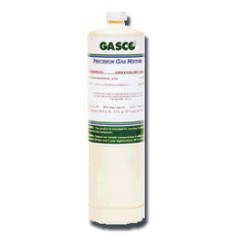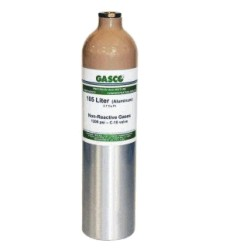Calibration gas blends, mixes and accessories
NON-REACTIVE SINGLE GAS > PROPANE :    24 Items found.(1580)

## NORLAB Equivalent  P1075400

Norlab Equivalent Calibration Gas 400 ppm Propane Balance air 17L Liter cylinder (Made by Gasco)

Configure

\$32.00(1581)

## NORLAB Equivalent  P107510

Norlab Equivalent Calibration Gas 10% LEL Propane (0.22% vol.) Balance air 17L Liter cylinder (Made by Gasco)

Configure

\$32.00(1582)

## NORLAB Equivalent  P107520

Norlab Equivalent Calibration Gas 20% LEL Propane (0.44% vol.) Balance air 17L Liter cylinder (Made by Gasco)

Configure

\$32.00(1583)

## NORLAB Equivalent  P107525

Norlab Equivalent Calibration Gas 25% LEL Propane (0.55% vol.) Balance air 17L Liter cylinder (Made by Gasco)

Configure

\$32.00(1584)

## NORLAB Equivalent  P107530

Norlab Equivalent Calibration Gas 30% LEL Propane (0.66% vol.) Balance air 17L Liter cylinder (Made by Gasco)

Configure

\$32.00(1585)

## NORLAB Equivalent  P107545

Norlab Equivalent Calibration Gas 45% LEL Propane (1.0% vol.) Balance air 17L Liter cylinder (Made by Gasco)

Configure

\$32.00(1586)

## NORLAB Equivalent  P107550

Norlab Equivalent Calibration Gas 50% LEL Propane (1.05% vol.) Balance air 17L Liter cylinder (Made by Gasco)

Configure

\$32.00(1359)

## NORLAB Equivalent  P19710162V

Norlab Equivalent Calibration Gas 50% LEL Propane Simulant (1.62% vol.) Balance air 17L Liter cylinder (Made by Gasco)

Configure

\$32.00(1590)

## NORLAB Equivalent  HP107520

Norlab Equivalent Calibration Gas 20% LEL Propane (0.44% vol.) Balance air 34LS Liter cylinder (Made by Gasco)

Configure

\$65.00(1403)

## NORLAB Equivalent  HP19710162V

Norlab Equivalent Calibration Gas 50% LEL Propane Simulant (1.62% vol.) Balance air 34LS Liter cylinder (Made by Gasco)

Configure

\$65.00(1589)

## NORLAB Equivalent  HP107510

Norlab Equivalent Calibration Gas 10% LEL Propane (0.22% vol.) Balance air 34LS Liter cylinder (Made by Gasco)

Configure

\$65.00(1591)

## NORLAB Equivalent  HP107525

Norlab Equivalent Calibration Gas 25% LEL Propane (0.55% vol.) Balance air 34LS Liter cylinder (Made by Gasco)

Configure

\$65.00(1592)

## NORLAB Equivalent  HP107530

Norlab Equivalent Calibration Gas 30% LEL Propane (0.66% vol.) Balance air 34LS Liter cylinder (Made by Gasco)

Configure

\$65.00(1593)

## NORLAB Equivalent  HP107545

Norlab Equivalent Calibration Gas 45% LEL Propane (1.0% vol.) Balance air 34LS Liter cylinder (Made by Gasco)

Configure

\$65.00(1594)

## NORLAB Equivalent  HP107550

Norlab Equivalent Calibration Gas 50% LEL Propane (1.05% vol.) Balance air 34LS Liter cylinder (Made by Gasco)

Configure

\$65.00(1588)

## NORLAB Equivalent  HP1075400

Norlab Equivalent Calibration Gas 400 ppm Propane Balance air 34LS Liter cylinder (Made by Gasco)

Configure

\$65.00(1602)

## NORLAB Equivalent  107550

Norlab Equivalent Calibration Gas 50% LEL Propane (1.05% vol.) Balance air 105L Liter cylinder (Made by Gasco)

Configure

\$97.20(1446)

## NORLAB Equivalent  19710162V

Norlab Equivalent Calibration Gas 50% LEL Propane Simulant (1.62% vol.) Balance air 105L Liter cylinder (Made by Gasco)

Configure

\$97.20(1596)

## NORLAB Equivalent  1075400

Norlab Equivalent Calibration Gas 400 ppm Propane Balance air 105L Liter cylinder (Made by Gasco)

Configure

\$97.20(1597)

## NORLAB Equivalent  107510

Norlab Equivalent Calibration Gas 10% LEL Propane (0.22% vol.) Balance air 105L Liter cylinder (Made by Gasco)

Configure

\$97.20
Page: *1 | 2 | All